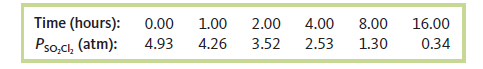# Problem: Sulfuryl chloride (SO2Cl2) decomposes to sulfur dioxide (SO2) and chlorine (Cl2) by reaction in the gas phase. The following pressure data were obtained when a sample containing 5.00 x 10-2 mol sulfuryl chloride was heated to 600. K in a 5.00 x 10 -1-L container.Defining the rate as - Δ [SO2Cl2] / Δt,a. determine the value of the rate constant for the decomposition of sulfuryl chloride at 600. K.

85% (46 ratings)
###### FREE Expert Solution

We're being asked to calculate for the rate constant for the decomposition of sulfuryl chloride at 600 K.

We don't know the order of the reaction. We will assume that the reaction is an elementary reaction which means that the given reaction is the slow step.

▪ the coefficients of the reactants in the slow step are the order of the reaction with respect to that reactant

Given reaction: Sulfuryl chloride (SO2Cl2) decomposes to sulfur dioxide (SO2) and chlorine (Cl2) by a reaction in the gas phase

SO2Cl2(g) → SO2(g) + Cl2(g)

85% (46 ratings)###### Problem Details

Sulfuryl chloride (SO2Cl2) decomposes to sulfur dioxide (SO2) and chlorine (Cl2) by reaction in the gas phase. The following pressure data were obtained when a sample containing 5.00 x 10-2 mol sulfuryl chloride was heated to 600. K in a 5.00 x 10 -1-L container.Defining the rate as - Δ [SO2Cl2] / Δt,
a. determine the value of the rate constant for the decomposition of sulfuryl chloride at 600. K.

Frequently Asked Questions

What scientific concept do you need to know in order to solve this problem?

Our tutors have indicated that to solve this problem you will need to apply the Integrated Rate Law concept. You can view video lessons to learn Integrated Rate Law. Or if you need more Integrated Rate Law practice, you can also practice Integrated Rate Law practice problems.

What is the difficulty of this problem?

Our tutors rated the difficulty ofSulfuryl chloride (SO2Cl2) decomposes to sulfur dioxide (SO2...as medium difficulty.

How long does this problem take to solve?

Our expert Chemistry tutor, Sabrina took 5 minutes and 25 seconds to solve this problem. You can follow their steps in the video explanation above.

What professor is this problem relevant for?

Based on our data, we think this problem is relevant for Professor Davidson's class at WEBER.

What textbook is this problem found in?

Our data indicates that this problem or a close variation was asked in Chemistry: An Atoms First Approach - Zumdahl Atoms 1st 2nd Edition. You can also practice Chemistry: An Atoms First Approach - Zumdahl Atoms 1st 2nd Edition practice problems.